# Forecasting Monthly Electric Production using ARIMA and Prophet (Part 1)

The Data of industrial production of electric and gas utilities in the United States were recorded from the years 1985–2018. This data measures the real output of all relevant establishments located in the United States, regardless of their ownership, but not those located in U.S. territories. We can find the data here.

How was the behavior of this monthly electric production data?

How about the electric production for the next 12 months?

There are several tools to analyze and get the model of time series data. In this article we’re gonna use two methods of time series analysis, ARIMA and Prophet. Before we’re going to the model and do forecasting, we want to get to know the data first.

The first step, import the library and data to be processed. There are 3 groups of libraries needed: the standard library for data processing and visualization, a library for stationary testing and time series analysis (ARIMA), and a library for calculating metrics when evaluating forecasting results. Then perform the initial visualization to see the behavior of the data.

`#Import Packagesimport pandas as pdfrom matplotlib import pyplot as plt #time series plotting# Ignore harmless warningsimport warningswarnings.filterwarnings("ignore")#ARIMA and SARIMA functionfrom statsmodels.graphics.tsaplots import plot_acffrom statsmodels.graphics.tsaplots import plot_pacffrom statsmodels.tsa.stattools import adfuller #stationary testfrom statsmodels.tsa.seasonal import seasonal_decomposefrom pmdarima import auto_arimafrom statsmodels.tsa.statespace.sarimax import SARIMAX# Calculating Metricsfrom sklearn.metrics import mean_squared_errorfrom statsmodels.tools.eval_measures import rmse#import datadf = pd.read_csv("Electric_Production.csv", parse_dates = ['DATE'], index_col = ['DATE'])df.head()`Dataset of Electric Production 1985–2018

We have two columns and 397 rows, the columns are DATE: beginning of each recorded month and IPG2211A2N: Industrial production index.

Next, we’re drawing the plot of the dataset.

`# Plot bulanan 1985-2018plt.figure(figsize=(11,10))plt.subplot(2, 1, 1)plt.plot(df)plt.axhline(y = df['IPG2211A2N'].mean(), color = 'r', linestyle = '-')plt.title('Image 1a. Monthly Electric Production (Jan 1985 - Jan 2018)')plt.xlabel('Date')plt.ylabel('Elec_Prod')plt.grid()plt.legend(['monthly observation','mean'], loc='upper left')# Plot bulanan 2010-2017start, end = '2010-01', '2017-12'plt.subplot(2, 1, 2)plt.plot(df.loc[start:end, 'IPG2211A2N'])plt.title('Image 1b. Monthly Electric Production (Jan 2010 - Dec 2017)')plt.xlabel('Date')plt.ylabel('Elec_Prod')plt.grid()plt.show()`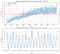Figure 1a and 1b. Monthly Electric Production 1985–2018 and 2010–2017

On Figure 1a, monthly electricity production data has an upward trend, especially from 1985 to 2008. The highest electricity production was in the most recent month of the recorded data, namely January 2018. In addition to the trend, if it is enlarged for data from 2010 to 2017, the data is suspected of having a seasonal pattern every 12 months. So that we enlarge the image for Jan 2010 to Dec 2017 on Figure 1b, we can clearly see that there is a similar data pattern every 12 months. Electricity production often drops on April and October, and often reaches its maximum value in January.

The stationarity of the data can be seen directly from the time observation plot, with the following characteristics:
1. There are no elements of seasonality and trends.
2. Has constant mean and variance properties.
3. The covariance between data is constant with each other (independent of time t).

From Figure 1a. Monthly Electric Production can be seen that the average is not constant, tends to increase or the trend is up. Then the variance of the data widened over time, the variance of the data in the 1980s was smaller than the data in the 2000s. In Figure 1b. that is, a sample of 2010–2017 data also found that the electricity production data has a seasonal pattern each year. From these conclusions it can be estimated that the Monthly Electricity Production data are not stationary.

However, it is more certain to use the stationary test, some of the tests that can be used are by looking visually through the ACF and PACF plots and precisely / numerically through the Augmented Dickey-Fuller (ADF) test. The hypothesis used:

If the p-value <0.05 then the null hypothesis is rejected or the data is stationary.

`def adf_test(dataset):    result = adfuller(dataset)    print('ADF Statistic: %f' % result)    print('p-value: %f' % result)    print('Critical Values:')    for key, value in result.items():        print('\t%s: %.4f' % (key, value))adf_test(df['IPG2211A2N'])`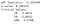ADF Test for Electric Production Data

From ADF test, it is obtained p-value = 0.1862 or p-value> 0.05 so that the null hypothesis is not rejected, or the data is not stationary. Data that are not stationary need to be differentiated to remove the trend effect. One-time differentiation results such as Image 4 below produce a more stationary value, there is no trend, the data is centered around the mean even though the variance is not constant enough. Apart from that, the seasonal effect is still visible in the data. The p-value <0.05 so that null hypothesis is rejected or the differentiated data is stationary.

`df2 = dfdf2['diff_1'] = df2['IPG2211A2N'].diff()df2.iloc[0,1] = 0adf_test(df2['diff_1'])`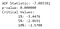ADF Test for Differentiated Data

We can see the plot of differentiated data compared to the original data. The trend already disappear but we can still see the seasonal pattern.

`# Plot bulanan 1985-2018plt.figure(figsize=(11,10))plt.subplot(2, 1, 1)plt.plot(df['IPG2211A2N'])plt.title('Image 4a. Monthly Electric Production (Jan 1985 - Jan 2018)')plt.xlabel('Date')plt.ylabel('Elec_Prod')plt.grid()# Plot Hasil Diferensiasiplt.subplot(2, 1, 2)plt.plot(df.IPG2211A2N.diff())plt.title('Image 4b. Differentiation of Monthly Electricity Production Data')plt.xlabel('Date')plt.ylabel('Diff_Elec_Prod')plt.grid()plt.show()`

Trend and seasonal patterns can also be seen from the ACF and PACF plots. ACF and PACF plot ACF or autocorrelation function is a function that describes the linear relationship between observations in time series analysis. In the ACF plot in Figure 3a, it can be seen that the data has a seasonal trend and pattern per 12 months. For Figure 4, namely the differentiated ACF and PACF data, there is no visible trend anymore, but the seasonal effect is still there.

`#Plot ACF dan PACF data asliplt.figure(figsize=(10,8))plt.subplot(211)plot_acf(df['IPG2211A2N'], ax=plt.gca())plt.title('Image 3a. ACF')plt.subplot(212)plot_pacf(df['IPG2211A2N'], ax=plt.gca())plt.title('Image 3b. PACF')plt.show()#Plot ACF dan PACF data hasil diferensiasiplt.figure(figsize=(10,8))plt.subplot(211)plot_acf(df2['diff_1'], ax=plt.gca())plt.title('Image 4a. ACF Hasil Diferensiasi 1')plt.subplot(212)plot_pacf(df2['diff_1'], ax=plt.gca())plt.title('Image 4b. PACF Hasil Diferensiasi 1')plt.show()`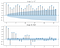ACF and PACF plot for Original Data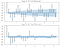ACF and PACF plot for Differentiated Data

# ARIMA (Auto-Regressive Integrated Moving Average)

To forecast data, first determine the time series model. The model is determined by the ARIMA (Autoregressive Integrated Moving Average) method which is a generalization of the ARMA (Autoregressive Moving Regressive) model, basically the ARIMA model is similar to linear regression. ARIMA consists of:

• AR (Autoregressive): A model that indicates the relationship between observation and past observation / observation lag.
• I (Integrated): The amount of differentiation made in the initial data
• MA (Moving Average): A model that uses the relationship between observations and errors from current and past observations.

There are three parameters of the ARIMA model, namely 𝑝: the observation lag of the AR model, 𝑑: a lot of differentiation, and q: the order of the moving average. So that the ARIMA model is often denoted by ARIMA (p, d, q).

Manually, the determination of the ARIMA model is done using the Box Jenkins Method:

1. Stationary test (differentiate to stationary).
2. Three iterative stages:
• Parameter identification (via ACF and PACF plots)
• Parameter estimate (with least squares, maximum likelihood)
• Diagnostic test (assuming normal distributed and uncorrelated residuals)

ARIMA model parameters are determined by looking at the ACF and PACF patterns. 𝐴𝑅 (𝑝) is when PACF is cut off after lag 𝑝 and ACF decreases sinusoidally. Whereas 𝑀𝐴 (𝑞), parameter 𝑞 is determined from the ACF plot which is cut off after lag 𝑞 and PACF decreases sinusoidally. From the identification of these parameters, several combinations of ARIMA models were obtained, then the ones with the minimum AIC value were selected.

For data that has a seasonal effect, the model used is Seasonal ARIMA or SARIMA (p, d, q) x (P, D, Q, s). This model adds parameters (P, D, Q, s) which are the parameters of the seasonal component.

Manual model determination takes longer, especially for seasonal data, because besides to differentiating to eliminate trends, another differentiation is necessary to eliminate seasonal factors. In addition, the determination of the parameter combination is seen visually first then the AIC is calculated. In Python, we can use the auto_arima function to find the best parameter combination more quickly, decided by the minimum AIC. Even though it is automatic, there are still parameters that need to be limited to prevent the function from running too long and prevent over-fitting. To determine the bounded parameters, refer to the ACF and PACF plots in Figures 3 and 4.

`# Pisahkan data train dan test, set data test untuk 1 tahun terakhirdf_train = df.iloc[:len(df)-12]df_test = df.iloc[len(df)-12:]# Fitting model ARIMA dengan algoritma stepwisestepwise_fit = auto_arima(df_train['IPG2211A2N'], start_p = 0, start_q = 0,                          m = 12, start_P = 0, D = 1,                           stationary = False, #diketahui tidak stasioner                          seasonal = True, #data punya efek musiman                          trace = True,                          error_action ='ignore',                             suppress_warnings = True,                           stepwise = True)           stepwise_fit.summary()`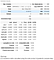Model determined by auto_arima function

The best model is obtained based on the AIC value for electricity production, namely SARIMA (1,1,1) x (2,1,1,12) or SARIMA (1,1,1) x (2,1,2,12). Both of them have an AIC that is not much different, which is around 1690. Furthermore, model fitting and evaluation of the two SARIMA models will be carried out to find a model with the smallest RMSE and MSE. Fitting is done with the SARIMAX function, which is an expansion model of SARIMA with the addition of an exogenous factor (optional). However, in this dataset, there are no exogenous factors, so the model remains SARIMA.

The results show that from the two models, the difference between MSE and RMSE is very small, but SARIMA (1,1,1) x (2,1,1,12) slightly smaller.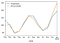Predictions of SARIMA (1,1,1)x(2,1,1,12)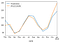Predictions of SARIMA (1,1,1)x(2,1,2,12)

We also checked the diagnostic test for SARIMA (1,1,1)x(2,1,1,12). A time series model is suitable if:

• Zero mean and constant residual variance
The residual plot is spread around zero without a trend.
• Residuals are normally distributed
The residual histogram is close to the red line of the normal distribution
• Residuals are independent of each other
The residual correlation value at each lag is within the limit of significance.

Based on diagnostic plot below, we could conclude residuals of SARIMA(1,1,1)x(2,1,1,12) have zero mean, constant variance, normally distributed, and independent. So, this model is suitable.

So, we choose the model for Electric Production is SARIMA (1,1,1)x(2,1,1,2). We fitted this model with the full Electric Production data then we obtained the coefficient for SARIMA equations.

`# Fitting model ke full datasetmodel = SARIMAX(df['IPG2211A2N'], order = (1, 1, 1), seasonal_order =(2, 1, 1, 12))result = model.fit()result.summary()`

Next, we can forecast Electric Production for the next 12 months using the model we obtained.

`# Forecast data untuk 12 bulan selanjutnyaforecast = result.predict(start = len(df), end = (len(df)-1) + 12, typ = ‘levels’).rename(‘Forecast’)df_forecast = pd.DataFrame(forecast)display(df_forecast)# Plot nilai hasil forecasting 12 bulan selanjutnyadf[‘IPG2211A2N’].plot(figsize = (12, 5), legend = True)forecast.plot(legend = True)plt.title(‘Image 5. Forecast Monthly Electric Production (Feb 2018 — Jan 2019)’)plt.xlabel(‘Date’)plt.ylabel(‘Elec_Prod’)plt.grid()plt.show()`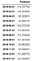Forecast of Electric Production for Feb 2018 — Jan 2019

The next part, we will try to forecast the data using time-series library from Facebook called Prophet.

Excited to learn more in data field, sometimes read, mostly watch movies.

## More from Dana Annisa Riefina

Excited to learn more in data field, sometimes read, mostly watch movies.